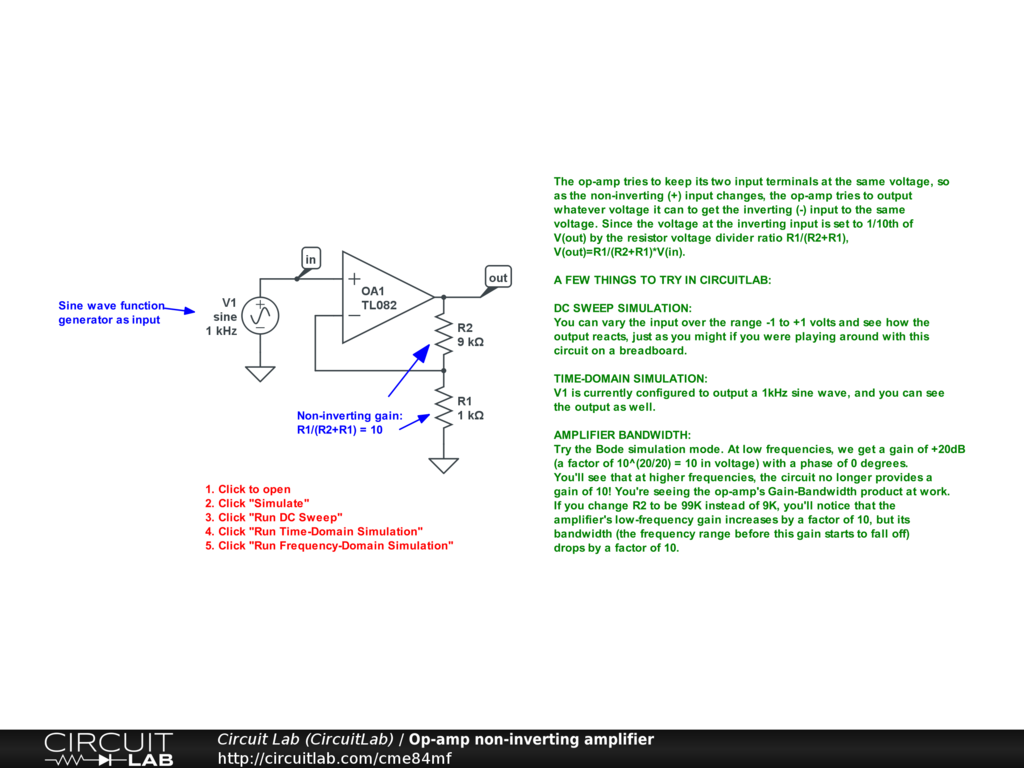Created by Created November 13, 2011 Last modified June 08, 2017 Tags

### Summary

Two resistors plus an op-amp form a gain-of-10 (inverted) amplifier.

### Description

The inverting (-) input of the op-amp forms a virtual ground because the op-amp tries to keep its two terminals at the same voltage. Since no current flows into the op-amp inputs, the current V1/R1 flows through R2, causing a voltage drop, so V(out) = -R2/R1*V(in).

#### A few things to try in CircuitLab

##### DC sweep simulation

You can vary the input over the range -1 to +1 volts and see how the output reacts, just as you might if you were playing around with this circuit on a breadboard.

##### Time simulation

V1 is currently configured to output a 1kHz sine wave, and you can see the output as well.

##### Amplifier bandwidth

Try the Bode simulation mode. At low frequencies, we get a gain of +20dB (a factor of 10^(20/20) = 10 in voltage) with a phase of -180 degrees -- this means -10. You'll see that at higher frequencies, the circuit no longer provides a gain of -10! You're seeing the op-amp's Gain-Bandwidth product at work. If you change R2 to be 100K instead of 10K, you'll notice that the amplifier's low-frequency gain increases by a factor of 10, but its bandwidth (the frequency range before this gain starts to fall off) drops by a factor of 10.

Op-amp non-inverting amplifier: